# Olympiad Test: Direction Sense Test -2

## 15 Questions MCQ Test Mathematical Olympiad Class 8 | Olympiad Test: Direction Sense Test -2

Description
Attempt Olympiad Test: Direction Sense Test -2 | 15 questions in 30 minutes | Mock test for Class 8 preparation | Free important questions MCQ to study Mathematical Olympiad Class 8 for Class 8 Exam | Download free PDF with solutions
QUESTION: 1

### It is 3 O clock in a watch. If the minute hand points towards the north-east then what is the direction of hour hand point?

Solution: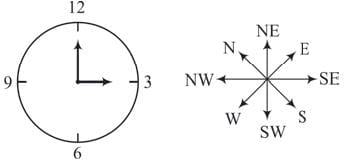QUESTION: 2

### Hari is 40m south-west of Rohit. Sukeah is to the east of Rohit. Then Sukeah is in which direction of Hari?

Solution: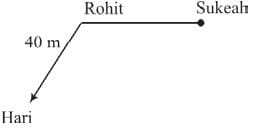Clearly Sukeah is in North-east direction of Hari.

QUESTION: 3

### Rata walked 30m towards south. Then he turn to his left and walked 25m. He then turned to his left and walked 30m. He again turned to his right and walked 35m. At what distance and in which direction is he from the starting point?

Solution: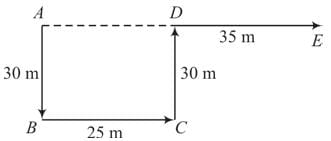AE = AD + DE = 25 + 35 = 60 m in East direction.

QUESTION: 4

Kishan goes 30m North then turns right and walked 50m, then again turns right and walks 20m then again turns right and walks 50m. What is the distance of new position from original position?

Solution: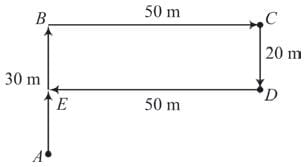Starting point = A
Ending point = E
Required distance AE = AB – BE
= 30 – 20 = 10 m

QUESTION: 5

Monaj is facing South. He turns 135° in the anticlock-wise direction and then 180° in the clock-wise direction. Which direction is he facing now?

Solution: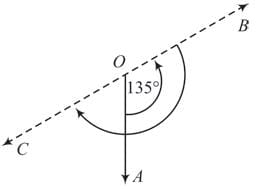Final direction of Manas = OC, which is in South-west.

QUESTION: 6

Rina walks 18m towards west then she turns to her right and walks 18m and then turns to her left and walks 13m. Again turning to her left and walked 18m. What is the shortest distance between her starting point and new position?

Solution: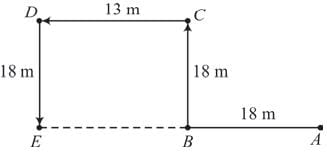Starting point = A, Ending point = E Required distance AE = AB + BE = 18 + 13 = 31 m

QUESTION: 7

From his house, Mihir went 15km to the north then he turned west and covered 10km. Then he turned south and covered 5km. Finally turning to east he covered 10km. In which direction is he from his house?

Solution: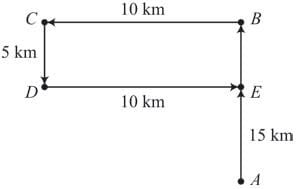Starting point = A,  Ending point = E

E is North from point A.

QUESTION: 8

Going 70m to the south of her house Madhu turns left and goes another 20m. Then she turns towards North and covers 40m and starts walking to her house. In which direction is she walking now?

Solution: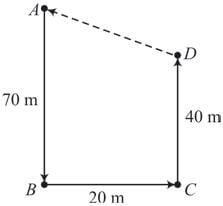Starting point = A,  Ending point = D
Final direction towards Madhu’s house = DA which is in North-West.

QUESTION: 9

Aman is facing North-West. He turns 90° in the clockwise direction and then 135° in the anti-clockwise direction. In which direction is he facing now?

Solution: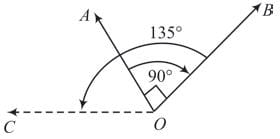O is starting position.
Finally Aman is facing toward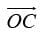which is in west.

QUESTION: 10

John went 15km to the west from my house then turned left and walked 20km. He then turned East and walked 25km. And finally turning left and walked 20km. How far is he from his house?

Solution: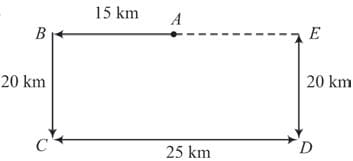Starting point = A
Ending point = E
AE = B E – AB
= 25 – 15 = 10 km

QUESTION: 11

A,B,C,D,E,F,G,H are sitting around a round table in the same order for group discussionat equal distances. Their positions are clockwise. If G sits in the North, then what will be the position of D?

Solution: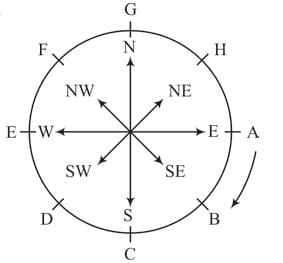In the above figure, position of D is southwest.

QUESTION: 12

Shankar wants to go to the college. He starts from his house which is in the east and covers to a crossing. The road to the left ends in a Hospital, straight ahead is the Library. In which direction is the college ?

Solution: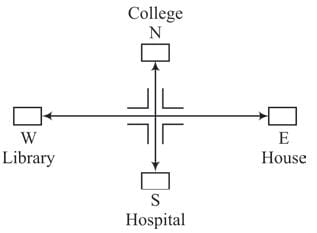College is in North direction.

QUESTION: 13

A watch reads 4:30. If the hour hand points towards North-east. In which direction the minute hand points?

Solution: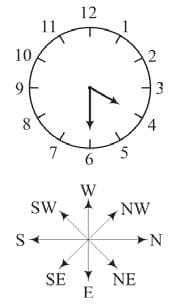Minute hand points ‘East’.

QUESTION: 14

If North is called North-West, South-West is called South, North-West is called west then what is East called?

Solution: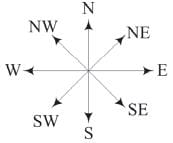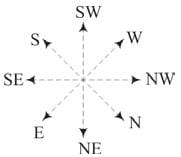QUESTION: 15

Lalit is facing west. He turns 45° in the clockwise direction and then another 180° in the same direction and then 270° in the anticlock wise direction. In which is he facing now?

Solution: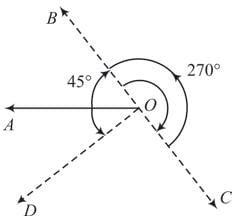Lalit’s starting position is O and his final position is D, which is in South-west direction.Use Code STAYHOME200 and get INR 200 additional OFF Use Coupon Code